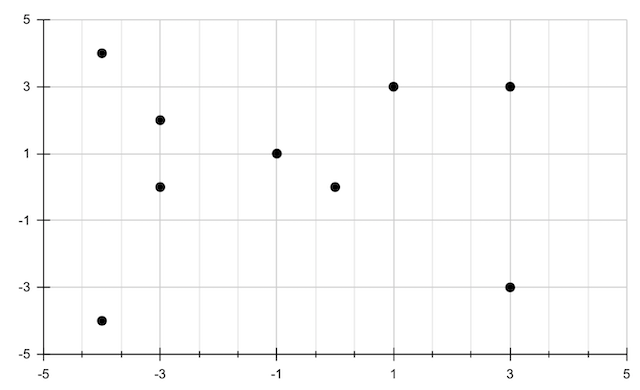## Lesson goal: Reasoning about points and lines

Previous: Converting units | Home | Next: Reasoning about points and lines (2)

Suppose we had a collection of $(x,y)$ points as shown here.Let's look at some Prolog code that will allow us to mathematically reason about these points. But what reasoning can we do about points? Well, to start, two points can form a line right? So we can look at lines that are possible to form by pairs of points.

Specifying points in easy in Prolog. They're pretty fundamental, so they're like facts. We can just type up some point(x,y). facts as you can see at the top of the code below, which describes the points in the above graph.

How about lines formed by the points? To start, let's have Prolog search through the points and tell us what lines are possible given the points. You can see this in the line([(X1,Y1),(X2,Y2)]) clause below. This clause says "find a point $(x_1,y_1)$ and another point $(x_2,y_2)$, which defines a line. Also be sure that the line's two points are not the same, $(x_1,y_1)\ne (x_2,y_2)$." (Our format for a line will look like this $[(x_1,y_2),(x_2,y_2)]$, a Prolog list of two $(x,y)$ points.) If we run line(L) for example, one result will be L = [(-4,4),(-3,2)] .

For some more reasoning, let's also see if Prolog can tell if lines it finds are horizontal and/or vertical line. How?

We know from math that a line is horizontal if it passes through two points whose $y$-coordinates are the same. You can see the horiz clause below, horiz([(_,Y),(_,Y)]). See how the Y is forced to be in common between the two points on the line (and we don't care about the $x$-coordinates).

Pretty similar for vertical lines: we look for points that have the same $x$-coordinate, as in vert([(X,_),(X,_)]), where you can note how the X coordinates are forced to be the same for both points of the line (and we don't care about the $y$-coordinates).